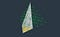# Notes for Teachers (American Lit.)

The Martian Math course gets us ready to read Industrial Poets, especially architecture pioneer R. Buckminster Fuller, latter day American Transcendentalist (grand nephew of Margaret, a founding mother of the movement).

phere volume = (4/3) pi r r r (or “r to the 3rd power”) is the conventional volume formula. r r r is imagined to be a cube of edges r (not a tetrahedron).

If an IVM tetrahedron is unit volume instead, figure out how it relates to the XYZ unit cube.

By convention, we choose a tetrahedron of twice the cube’s edges, which makes for only a 6% difference (the tetrahedron is a tad smaller, even with the longer edges).

The Sphere Volume is therefore bigger (in terms of the smaller unit) by a factor of about 1.06. Algebraically, we can write it as √2 pi r r r.(2/5) x (2/5) x 1 = 4/25 of Unit; 2 x 2 x 5 = 20 of 5 to the 3rd

In the IVM, a rhombic dodecahedron cell, container of said Sphere of unit radius, has volume 6, so sphere to empty space density is: √2 pi/6 or ~0.74048.

That agrees with the XYZ answer, as it should, as we’re talking volume ratios, so the unit cancels out (just be consistent with your denominations (your currencies)).

igh schoolers learning recent American lit have gotta learn this stuff. Math majors are off the hook.

The XYZ apparatus features cubes packed together, sharing edges, of unit volume. The IVM is a similar apparatus, studied by Alexander Graham Bell as a structure, and known to crystallographers as the FCC and CCP, in their talking about vertex placement.

The rods interconnecting these vertexes form a skeleton of tetrahedrons and octahedrons. Taking the tetrahedrons as unit makes the octahedrons volume 4. The rhombic cells surrounding each sphere have volume 6 (in tetravolumes).

Denominating polyhedrons in tetrahedrons, is what Synergetics is all about.

Like a different currency. But there’s a conversion constant. 2nd root of 9/8.

Written by